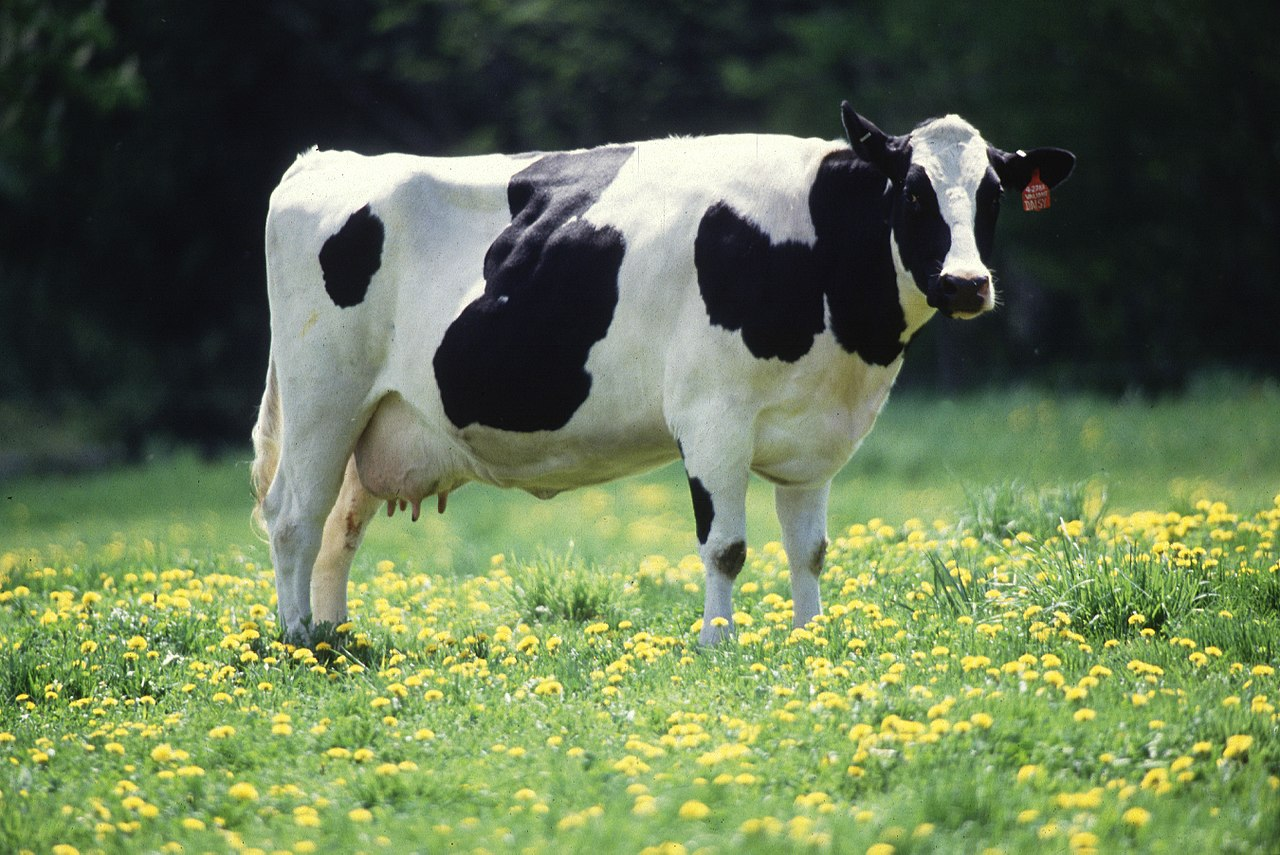# Why we broke your PR

As some of you probably noticed a lot of PRs on the backlog now need a rebase because of PR 20137. This post is to explain why.

Godot has a copy on write or 'CoW' feature for a lot of its data structures. What this means in practice is basically that:

``````Vector<int> vec1;
for (int i = 0; i < MAX_INT; ++i) {
vec1.push_back(i);
}
Vector<int> vec2 = vec1;``````

Is basically free, vec1 and vec2 will share memory and this basically takes no time. Also please don't write this code :).

Of course someone may take a a copy of a vector and modify it, or the original owner of the vector may want to write to it. At this point CoW comes into play. As soon as a write happens to either vec1 or vec2 we copy the contents and make the vectors unique.

The problem is that in the previous implementation of Vector this no longer worked. When Reduz implemented this compilers were able to deduce what overload to call based on the return type of a method. This is no longer possible so all calls to vector elements actually caused a copy on write. So we were basically doing a 'copy on read' instead of a 'copy on write'. Greatly increasing the cost of a read.

This has now been fixed. So the above code still works as-is:

``````Vector<int> vec1;
for (int i = 0; i < MAX_INT; ++i) {
vec1.push_back(i);
}
Vector<int> vec2 = vec1;``````

However now the following no longer compiles

``vec1 = 20;``

``vec1.write = 20;``